## Subspaces ofRn

Consider the collection of vectorsThe endpoints of all such vectors lie on the line y = 3 x in the x‐y plane. Now, choose any two vectors from V, say, u = (1, 3) and v = (‐2, ‐6). Note that the sum of u and v,is also a vector in V, because its second component is three times the first. In fact, it can be easily shown that the sum of any two vectors in V will produce a vector that again lies in V. The set V is therefore said to be closed under addition. Next, consider a scalar multiple of u, say,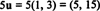It, too, is in V. In fact, every scalar multiple of any vector in V is itself an element of V. The set V is therefore said to be closed under scalar multiplication.

Thus, the elements in V enjoy the following two properties:

The sum of any two elements in V is an element of V.

Closure under scalar multiplication:

Every scalar multiple of an element in V is an element of V.

Any subset of R n that satisfies these two properties—with the usual operations of addition and scalar multiplication—is called a subspace of Rn or a Euclidean vector space. The set V = {(x, 3 x): xR} is a Euclidean vector space, a subspace of R2.

Example 1: Is the following set a subspace of R2?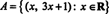To establish that A is a subspace of R2, it must be shown that A is closed under addition and scalar multiplication. If a counterexample to even one of these properties can be found, then the set is not a subspace. In the present case, it is very easy to find such a counterexample. For instance, both u = (1, 4) and v = (2, 7) are in A, but their sum, u + v = (3, 11), is not. In order for a vector v = (v 1, v 2 to be in A, the second component (v 2) must be 1 more than three times the first component (v 1). Since 11 ≠ 3(3) + 1, (3, 11) ∉ A. Therefore, the set A is not closed under addition, so A cannot be a subspace. [You could also show that this particular set is not a subspace of R 2 by exhibiting a counterexample to closure under scalar multiplication. For example, although u = (1, 4) is in A, the scalar multiple 2 u = (2, 8) is not.]

Example 2: Is the following set a subspace of R3?In order for a sub set of R 3 to be a sub space of R 3, both closure properties (1) and (2) must be satisfied. However, note that while u = (1, 1, 1) and v = (2, 4, 8) are both in B, their sum, (3, 5, 9), clearly is not. Since B is not closed under addition, B is not a subspace of R 3.

Example 3: Is the following set a subspace of R4?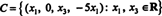For a 4‐vector to be in C, exactly two conditions must be satisfied: Namely, its second component must be zero, and its fourth component must be −5 times the first. Choosing particular vectors in C and checking closure under addition and scalar multiplication would lead you to conjecture that C is indeed a subspace. However, no matter how many specific examples you provide showing that the closure properties are satisfied, the fact that C is a subspace is established only when a general proof is given. So let u = (u1, 0, u3, −5 u1) and v = (v1, 0, v3, −5v1) be arbitrary vectors in C. Then their sum,satisfies the conditions for membership in C, verifying closure under addition. Finally, if k is a scalar, thenis in C, establishing closure under scalar multiplication. This proves that C is a subspace of R4.

Example 4: Show that if V is a subspace of R n, then V must contain the zero vector.

First, choose any vector v in V. Since V is a subspace, it must be closed under scalar multiplication. By selecting 0 as the scalar, the vector 0 v, which equals 0, must be in V. [Another method proceeds like this: If v is in V, then the scalar multiple (−1) v = − v must also be in V. But then the sum of these two vectors, v + (− v) = 0, mnust be in V, since V is closed under addition.]

This result can provide a quick way to conclude that a particular set is not a Euclidean space. If the set does not contain the zero vector, then it cannot be a subspace. For example, the set A in Example 1 above could not be a subspace of R 2 because it does not contain the vector 0 = (0, 0). It is important to realize that containing the zero vector is a necessary condition for a set to be a Euclidean space, not a sufficient one. That is, just because a set contains the zero vector does not guarantee that it is a Euclidean space (for example, consider the set B in Example 2); the guarantee is that if the set does not contain 0, then it is not a Euclidean vector space.

As always, the distinction between vectors and points can be blurred, and sets consisting of points in Rn can be considered for classification as subspaces.

Example 5: Is the following set a subspace of R2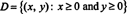As illustrated in Figure , this set consists of all points in the first quadrant, including the points (x, 0) on the x axis with x > 0 and the points (0, y) on the y axis with y > 0:Figure 1

The set D is closed under addition since the sum of nonnegative numbers is nonnegative. That is, if (x1, y1) and (x2, y2) are in D, then x1, x2, y1, and y2 are all greater than or equal to 0, so both sums x1 + x2 and y1 + y2 are greater than or equal to 0. This implies that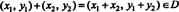However, D is not closed under scalar multiplication. If x and y are both positive, then ( x, y) is in D, but for any negative scalar k,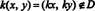since kx < 0 (and ky < 0). Therefore, D is not a subspace of R 2.

Example 6: Is the following set a subspace of R 2?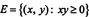As illustrated in Figure , this set consists of all points in the first and third quadrants, including the axes:Figure 2

The set E is closed under scalar multiplication, since if k is any scalar, then k(x, y) = ( kx, ky) is in E. The proof of this last statement follows immediately from the condition for membership in E. A point is in E if the product of its two coordinates is nonnegative. Since k 2 > 0 for any real k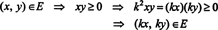However, although E is closed under scalar multiplication, it is not closed under addition. For example, although u = (4, 1) and v = (−2, −6) are both in E, their sum, (2, −5), is not. Thus, E is not a subspace of R 2.

Example 7: Does the plane P given by the equation 2 x + y − 3 z = 0 form a subspace of R 3?

One way to characterize P is to solve the given equation for y,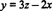and writeIf p 1 = ( x 1, 3 z 1 − 2 x 1, z 1) and p 2 = ( x 2, 3 z 2 − 2 x 2, z 2) are points in P, then their sum,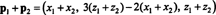is also in P, so P is closed under addition. Furthermore, if p = ( x, 3 z − 2 x, z) is a point in P, then any scalar multiple,is also in P, so P is also closed under scalar multiplication. Therefore, P does indeed form a subspace of R 3. Note that P contains the origin. By contrast, the plane 2 x + y − 3 z = 1, although parallel to P, is not a subspace of R 3 because it does not contain (0, 0, 0); recall Example 4 above. In fact, a plane in R 3 is a subspace of R 3 if and only if it contains the origin.